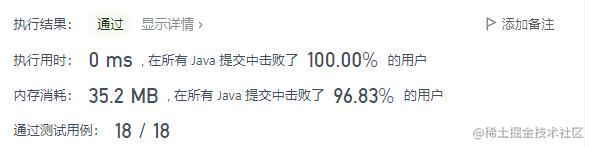leetcode刷题n23-q168：Excel表列名称

题目描述

A -> 1 B -> 2 C -> 3 ... Z -> 26 AA -> 27 AB -> 28 ...

1 <= columnNumber <= 231 - 1

思路 & CODE

1. 26进制运算

[A-Z]对应[1-26]，范围相当于是1*26^0 ~ 26 * 26 ^ 0

[AA-ZZ]对应[27-702],范围相当于是26*26^0 + 0*26^1 ~ 26*26^0 + 26*26^1

a1 * 26^0 + a2 * 26^1 + a3 * 26^2 + ... = n

n = a1

a2 * 26^0 + a3 * 26^1 + ... = n

• 例子

public String convertToTitle(int columnNumber) {
StringBuilder res = new StringBuilder();

while (columnNumber > 0) {
columnNumber--;
int mod = columnNumber % 26;
res.append((char) (mod + 'A'));
columnNumber /= 26;
}
return res.reverse().toString();
}